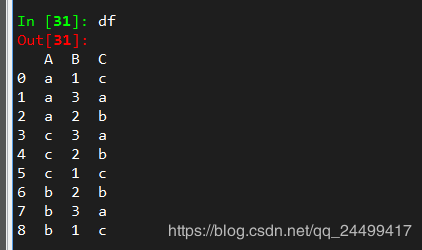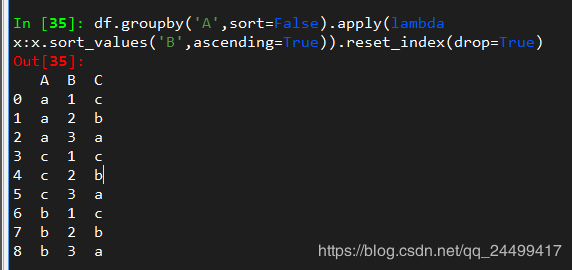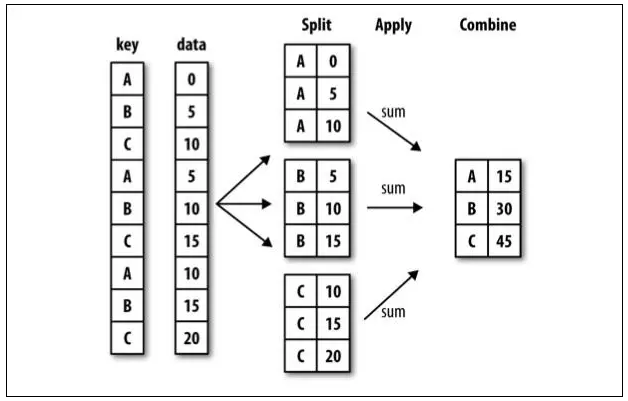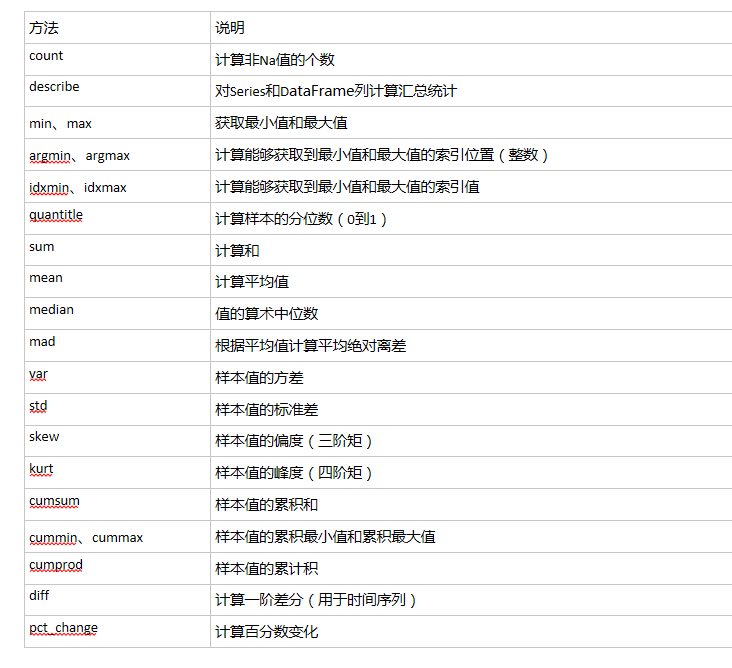• 文章转载：https://blog.csdn.net/qq_24499417/article/details/87265931...需要对df进行分组排序。 import pandas as pd df = pd.DataFrame([['a', 1, 'c'], ['a', 3, 'a'], ['a', 2, 'b'], ['c', 3, 'a'], ['c'...
文章转载：https://blog.csdn.net/qq_24499417/article/details/87265931
需要对df进行分组后排序。
import pandas as pd
df = pd.DataFrame([['a', 1, 'c'], ['a', 3, 'a'], ['a', 2, 'b'],
['c', 3, 'a'], ['c', 2, 'b'], ['c', 1, 'c'],
['b', 2, 'b'], ['b', 3, 'a'], ['b',1, 'c']], columns=['A', 'B', 'C'])按照A列进行分组后对B列进行升序排序：展开全文python
• 一、pandas分组* 1、分组运算过程：split->apply->combine 拆分：进行分组的根据 应用：每个分组运行的计算规则 ...DataFrame.groupby(by=None, axis=0, level=None, as_index=True, sort=True, group_keys=Tr.
转载自 https://blog.csdn.net/ai_1046067944/article/details/86300634
一、pandas分组*
1、分组运算过程：split->apply->combine
拆分：进行分组的根据应用：每个分组运行的计算规则合并：把每个分组的计算结果合并起来2、分组函数
DataFrame.groupby(by=None, axis=0, level=None, as_index=True, sort=True, group_keys=True, squeeze=False, observed=False, **kwargs

by： 依据哪些列进行分组，值可以是：mapping, function, label, or list of labels

3、聚合函数4、分组聚合实例
单列分组
>>> import pandas as pd

>>> df = pd.DataFrame({'A': ['a', 'b', 'a', 'c', 'a', 'c', 'b', 'c'], 'B': [2, 8, 1, 4,

3, 2, 5, 9], 'C': [102, 98, 107, 104, 115, 87, 92, 123], 'D': [2, 98, 17, 14, 15, 7, 92,

13]})

>>> df

A  B    C   D

0  a  2  102   2

1  b  8   98  98

2  a  1  107  17

3  c  4  104  14

4  a  3  115  15

5  c  2   87   7

6  b  5   92  92

7  c  9  123  13

>>> df.groupby(by='A').sum()

B    C    D

A

a   6  324   34

b  13  190  190

c  15  314   34

多列分组
>>> df.groupby(by=['A','B']).sum()             ###A,B成索引

C   D

A B

a 1  107  17

2  102   2

3  115  15

b 5   92  92

8   98  98

c 2   87   7

4  104  14

9  123  13

多列聚合
>>> df.groupby(by=['A','B'])['C'].sum()      ###1个列

A  B

a  1    107

2    102

3    115

b  5     92

8     98

c  2     87

4    104

9    123

>>> df.groupby(by=['A','B'])['C','D'].sum()   ###2个列

C   D

A B

a 1  107  17

2  102   2

3  115  15

b 5   92  92

8   98  98

c 2   87   7

4  104  14

9  123  13

多列不同聚合方式
>>> import numpy as np

>>> df.groupby(by=['A']).agg({'C':[np.mean, 'sum'], 'D':['count',np.std]})

C          D

mean  sum count       std

A

a  108.000000  324     3  8.144528

b   95.000000  190     2  4.242641

c  104.666667  314     3  3.785939

>>>ps: 不同列使用多个不同函数进行聚合C： mean，sum；D：count，std

返回值类型区别
方法1：agg

>>> df.groupby(by=['A']).agg({'C':[np.mean]})

C

mean

A

a  108.000000

b   95.000000

c  104.666667

>>> type(df.groupby(by=['A']).agg({'C':[np.mean]}))

<class 'pandas.core.frame.DataFrame'>

方法2：索引

>>> df.groupby(by=['A'])['C'].mean()

A

a    108.000000

b     95.000000

c    104.666667

Name: C, dtype: float64

>>> type(df.groupby(by=['A'])['C'].mean())

<class 'pandas.core.series.Series'>

总结： 两种方法结果一样，但是一个类型是DataFrame,一个为Series；有时候会用上

二、pandas排序
按索引进行降序排列
>>> df

A  B    C   D

0  a  2  102   2

1  b  8   98  98

2  a  1  107  17

3  c  4  104  14

4  a  3  115  15

5  c  2   87   7

6  b  5   92  92

7  c  9  123  13

>>> df.sort_index(ascending=False)       ### 索引

A  B    C   D

7  c  9  123  13

6  b  5   92  92

5  c  2   87   7

4  a  3  115  15

3  c  4  104  14

2  a  1  107  17

1  b  8   98  98

0  a  2  102   2

按值进行降序排列
>>> df.sort_values(by="A",ascending=False)        # 按某一列

A  B    C   D

3  c  4  104  14

5  c  2   87   7

7  c  9  123  13

1  b  8   98  98

6  b  5   92  92

0  a  2  102   2

2  a  1  107  17

4  a  3  115  15

>>> df.sort_values(by=["B","A"],ascending=False)   # 按2列

A  B    C   D

7  c  9  123  13

1  b  8   98  98

6  b  5   92  92

3  c  4  104  14

4  a  3  115  15

5  c  2   87   7

0  a  2  102   2

2  a  1  107  17


展开全文python pandas
• Python Dataframe 分组排序和 Modin 1、按照其中一列进行排序 在dataframe中，按照其中的一列排序：比如q值倒排 (1)rank方法 data['new_rank'] = data.groupby('house_code')['q_score_new'].rank(ascending=...
Python Dataframe 分组排序和 Modin

1、按照其中一列进行排序
在dataframe中，按照其中的一列排序：比如q值倒排
(1)rank方法
data['new_rank'] = data.groupby('house_code')['q_score_new'].rank(ascending=False, method='dense')
(2)sort_values方法
data.sort_values(['q_score_new'], ascending=False).groupby(['house_code']).cumcount() + 1 
2、按照其中多列进行排序
在dataframe中，按照其中的多列排序：比如q值倒排、经纪人ucid正排
sort_values方法
(1)dsort_values方法ata.sort_values(['q_score_new', 'agent_ucid'], ascending=[ False, True]).groupby(['house_code']).cumcount() + 1 # 20多秒（100多万行数据集上）

(2)data = data.groupby('house_code').sort_values(by=['q_score_new', 'agent_ucid'], ascending=(False, True)).groupby('house_code').apply(f) # 200多秒（100多万行数据集上）

def f(df):
df['new_rank'] = range(1, len(df) + 1)
return df
最终结果显示：第一种比第二种（）快10倍左右（在200万行数据集上），所以推荐第二种！！！
3、modin
(1)简介
Modin 是加州大学伯克利分校 RISELab 的一个早期项目，旨在促进分布式计算在数据科学领域的应用。它是一个多进程的数据帧（Dataframe）库，具有与 Pandas 相同的应用程序接口（API），使用户可以加速他们的 Pandas 工作流。
(2)原理
从本质上讲，Modin 所做的只是增加了 CPU 所有内核的利用率，从而提供了更好的性能。
(3)安装使用
Modin 是完全开源的，可以通过下面的 GitHub 链接获得：
https://github.com/modin-project/modin
我们可以使用如下所示的 PyPi 指令来安装 Modin：
pip install modin
在 Windows 环境下，Ray 是安装 Modin 所需的依赖之一。Windows 本身并不支持 Ray，所以为了安装它，用户需要使用 WSL（适用 Linux 的 Windows 子系统，适用Linux和mac）
(4)性能提升
pandas的内置函数（比如groupby等）因为优化的很好，所以和modin.pandas处理速度差不多，提升主要提现在数据读取上。 通常，Modin 使用「read_csv」函数读取 2G 数据需要 2 秒，而 读取 18G 数据大约需要不到 18 秒。
%%time
import pandas
-----------------------------------------------------------------
CPU times: user 26.3 s, sys: 3.14 s, total: 29.4s
Wall time: 29.5 s
%%time
import modin.pandas
-----------------------------------------------------------------
CPU times: user 76.7 ms, sys: 5.08 ms, total: 81.8 ms
Wall time: 7.6 s
(5)未来
Modin 项目仍处于早期阶段，但对 Pandas 来说是一个非常有发展前景的补充。Modin 为用户处理所有的数据分区和重组任务，这样我们就可以集中精力处理工作流。Modin 的基本目标是让用户能够在小数据和大数据上使用相同的工具，而不用考虑改变 API 来适应不同的数据规模
展开全文• Python 对DataFrame数据分组排序并选择最优数据 目的：对数据按第一列分组，按第二列降序排序，按第三列升序排序，选择每组中最好的输出 数据： 开始： import pandas as pd df = pd.read_excel('product.xlsx',...
• 之前分享过在SQL里面进行分组排序的方法，这里分享一下Python对DataFrame进行分组排序的方法。 假设我们有以下数据： ID Data 01 100 01 200 01 300 02 100 02 200 分组排序方法： df['rank'] = ...
之前分享过在SQL里面进行分组排序的方法，这里分享一下Python对DataFrame进行分组排序的方法。 假设我们有以下数据：
IDData0110001200013000210002200
分组排序方法：
df['rank'] = df['Data'].groupby(df['ID']).rank()

执行结果如下：
IDDatarank011001.0012002.0013003.0021001.0022002.0
谢谢观看，喜欢请点赞，谢谢！！！
展开全文Python rank
• 需要对df进行分组排序 import pandas as pd df = pd.DataFrame([['a', 100, 'c'], ['a', 300, 'a'], ['a', 200, 'b'], ['c', 300, 'a'], ['c', 200, 'b'], ['c', 100, 'c'], ['b', 200, 'b'], ['b', 300, 'a']...pandas python
• DataFrame ( data = data_dict ) df 2. 按班级分组 df = df . groupby ( 'class' , sort = False ) \ . apply ( lambda x : x . sort_values ( "score" , ascending = False ) ) \ . reset_index ...Pandas groupby
• 可以用df=df.fillna(0)直接填充相应的数值，也可以填充均值、中位数等值，具体操作如下（待补充）
• 准备dataset： import pandas as pd sql="select * from uds.amz_daily_sales where purchase_date&...1.排序 (1)单列 mysql: select * fromuds.amz_daily_saleswhere purchase_date>='2021-04-01' order b...python
• 使用dataframe解决spark TopN问题：分组排序、取TopN和join相关问题
• dataframe有两列，一列是分组依据，名称为id，另一列是cnt，需求是以id分组之后，删掉分组的cnt之和小于10的组。 首先拷贝一下dataframe： df1=df 然后对df1处理，设置新的index： df1=df1.set_index('id') 别...
• python的pandas包提供的数据聚合与分组运算功能很强大，也很灵活，本文就带领大家一起来了解groupby技术，感兴趣的朋友跟随小编一起来看下
• ## 只适合分组之后的一列排序，不能进行多列排序，更不能多列正逆序 ## 非数字列不能进行排序 ## 非数字列在numeric_only=False下，不能使用method='first',shit! ## pandas 2: pdf['row_num'] = pdf.sort_values(['...
• Dataframe分组统计group by函数 对数据进行分组统计主要使用Dataframe函数，其功能如下： 根据给定的条件将数据拆分成组。 每个组都可单独应用函数（如sum、mean、std等）。 将结果合并到一个数据结果中。 语法...python 数据分析
• pandas groupby 分组取每组的前几行记录方法，具有很好的参考价值，希望对大家有所帮助。 import pandas as pd df = pd.DataFrame({'class':['a','a','b','b','a','a','b','c','c'],'score':[3,5,6,7,8,9,10,11,14]}...
• 求教各位大神： 本人用scalas+spark开发，用RDD实现以下需求时遇到困难！ 数据： 用户 位置 天数 user1 L1 28 user1 L2 20 ...这里主体是根据用户分组计算最大天数，并把位置带出来，研究半天无果，求大神指教rdd spark
• SparkTools} import com.dhd.comment.Constant import com.profile.comment.Comments /** * 测试类 //使用dataframe解决spark TopN问题：分组排序、取TopN * @author * date 2021-11-27 14:55 */ object Test { ...spark scala 大数据
• Spark实现分组排序取topN 读取文件： Chinese zhangsan 90 Chinese lisi 80 Chinese wangwu 70 Math zhangsan 91 Math lisi 90 Math wangwu 95 English zhangsan 81 English lisi 82 English wangwu 83 代码实现： ...spark
• #创建两个dataframe t1=pd.DataFrame(np.random.randn(3,4),index=list("ABC"),columns=list("wxyz")) t2=pd.DataFrame(np.random.randn(2,6),index=list("AB"),columns=list("EFGHIJ")) t1,t2 # 默认how=left 即...python 数据分析
• 转载，侵删，感谢原作者 利用pandas做分组聚合时，分组组名默认是变化成index，如图 grouped = tdf.groupby...代码中tdf是一个dataframe当以uid分组，并求每组的平均值后在转化为dataframe,组名并入到index 这...
• DataFrame dataset = engine.EagerEvaluate("myDataFrame").AsDataFrame(); dataGridView_DF.DataSource = dataset; public enum IrisSpecies { setosa = 1, versicolor = 2, virginica = 3, } ...
• pandas组内排序，并在每个分组内按序打上序号 pandas dataframe 对dep_id组内的salary排序。希望给下面原本只有前三列的dataframe，添加上第四列。 等价于sql的row_number() 假设我已经建好了仅有前三列的dataframe...python
• 1.python 中分组统计 1.1按性别统计出年龄最大，最小，平均值 import pandas as pd df = pd.read_excel(r'./data.xlsx') print(df) ages = df.groupby(['gender'])['age'] ages_min = ages.min() ages_max = ...
• 实现dataframe某一列相等的行的其他列的聚合（分组） groupby分组后，通过apply(list)可以把column_index（1个值）对应的column_1（1个或多个值）变为list格式，输出为len(column_index)个( 一个index+1个list)的...
• 输入： 输出： 具体代码： ...data=pd.DataFrame({'c1':[5,8,3,3,4,1]}) print(data) d1= data.sort_values(by='c1') d1['rank']=d1.rank(method='min').astype(int) print(d1) 二：pyspa...python pyspark sql...